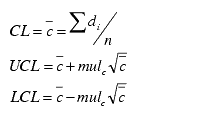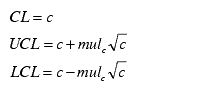# C chart calculations

C chart control limits are calculated as follows:

 Control Limits calculated from data Control Limits calculated from standard (mean of fraction of nonconformity ( c ) is given)## Descriptive Statistics

• Lower Control Limit (LCL)

• Upper Control Limit (UCL)

• Center Line (CL)